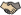Muzyka

Discussion in 'Arts, Sports, & Entertainment' started by Toroid, Jul 18, 2016.

•Like x 1
•Thanks x 1
1.
•Like x 2
2.
•Like x 2
3.
•Thanks x 1
•Like x 2
4.
•Thanks x 1
5.
•Thanks x 1
6.
•Thanks x 1
7.
•Thanks x 1
8.
•Thanks x 1
9.
•Thanks x 1
10.
•Like x 1
•Thanks x 1
11.
•Like x 1
•Thanks x 1
•Thanks x 1
•Like x 1
12.
•Thanks x 1
13.
•Thanks x 1
14.
•Thanks x 1
15.

16. Glory to the King